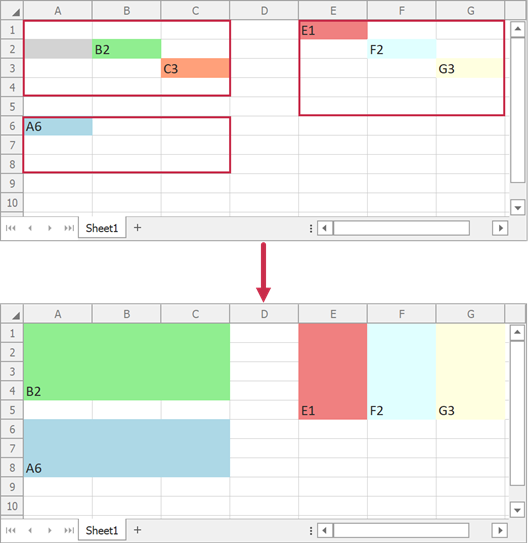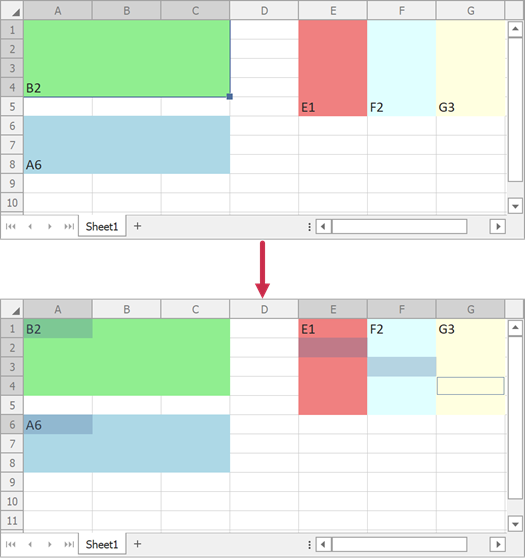.NET Framework 4.5.2+

# How to: Merge Cells or Split Merged Cells

This topic demonstrates how to merge a cell range into one cell and split merged cells.

## Merge Cells

To merge multiple adjacent cells into one cell, call the worksheet’s Worksheet.MergeCells method and pass the cell range you want to merge. You can also use the range’s RangeExtensions.Merge method to merge cells in this range.

Both methods can accept a MergeCellsMode parameter that allows you to specify how to merge cells (ignore or include intersections with other merged cells, merge rows, or merge columns). See the MergeCellsMode enumeration description for additional information and examples.

Note

When you merge a cell range, the data (value, formula, and format settings) of the top left non-empty cell appears in the resulting merged cell. Other cell data is lost.

If all cells within the original range are empty, the range’s top left cell format is applied to the merged cell.

In the following code sample, the Worksheet.MergeCells method merges the “A1:C4” cell range and the RangeExtensions.Merge method merges the “A6:C8” cell range into one cell and merges each column in the “E1:G5” cell range:``````using DevExpress.Spreadsheet;
using System.Drawing;
// ...

// Create a new Workbook object.
using (Workbook workbook = new Workbook())
{
// Access the first worksheet of the workbook.
Worksheet worksheet = workbook.Worksheets;

// Fill the "A2" cell with the LightGray color.
worksheet.Cells["A2"].FillColor = Color.LightGray;

// Specify the "B2" cell value.
worksheet.Cells["B2"].Value = "B2";
// Fill the "B2" cell with the LightGreen color.
worksheet.Cells["B2"].FillColor = Color.LightGreen;

// Specify the "C3" cell value.
worksheet.Cells["C3"].Value = "C3";
// Fill the "C3" cell with the LightSalmon color.
worksheet.Cells["C3"].FillColor = Color.LightSalmon;

// Specify the "A6" cell value.
worksheet.Cells["A6"].Value = "A6";
// Fill the "A6" cell with the LightBlue color.
worksheet.Cells["A6"].FillColor = Color.LightBlue;

// Specify the "E1" cell value.
worksheet.Cells["E1"].Value = "E1";
// Fill the "E1" cell with the LightCoral color.
worksheet.Cells["E1"].FillColor = Color.LightCoral;

// Specify the "F2" cell value.
worksheet.Cells["F2"].Value = "F2";
// Fill the "F2" cell with the LightCyan color.
worksheet.Cells["F2"].FillColor = Color.LightCyan;

// Specify the "G3" cell value.
worksheet.Cells["G3"].Value = "G3";
// Fill the "G3" cell with the LightYellow color.
worksheet.Cells["G3"].FillColor = Color.LightYellow;

// Merge the "A1:C4" cell range into one cell.
worksheet.MergeCells(worksheet.Range["A1:C4"]);

// Merge the "A6:C8" cell range into one cell.
worksheet.Range["A6:C8"].Merge();

// Merge the "E1:G5" cell range by columns.
worksheet.Range["E1:G5"].Merge(MergeCellsMode.ByColumns);
}
``````

## Split Merged Cells

Call the worksheet’s Worksheet.UnMergeCells method and pass the range that contains the merged cells. You can also use the range’s RangeExtensions.UnMerge method to split merged cells in this range.

The following code sample splits cells merged in the previous section:``````using DevExpress.Spreadsheet;
// ...

// Create a new Workbook object.
using (Workbook workbook = new Workbook())
{
// Access the first worksheet of the workbook.
Worksheet worksheet = workbook.Worksheets;
//...

// Split merged cells in the "A1:C4" cell range.
worksheet.UnMergeCells(worksheet.Range["A1:C4"]);

// Split merged cells in the "A6:C8" cell range.
worksheet.Range["A6:C8"].UnMerge();

// Split merged cells in the "E1:G5" cell range.
worksheet.Range["E1:G5"].UnMerge();
}
``````

### Split All Merged Cells in a Worksheet

Call the CellRange.GetMergedRanges method to obtain all merged ranges that intersect a specific area.

The following code sample finds and splits all merged cell ranges in a worksheet:

``````using DevExpress.Spreadsheet;
// ...

// Create a new Workbook object.
using (Workbook workbook = new Workbook())
{
// Access the first worksheet of the workbook.
Worksheet worksheet = workbook.Worksheets;
//...

// Split all merged cells in the worksheet.
foreach (var item in worksheet.Cells.GetMergedRanges()) {
item.UnMerge();
}
}
``````